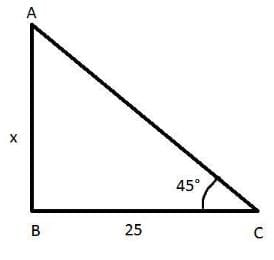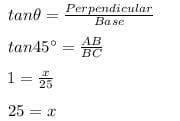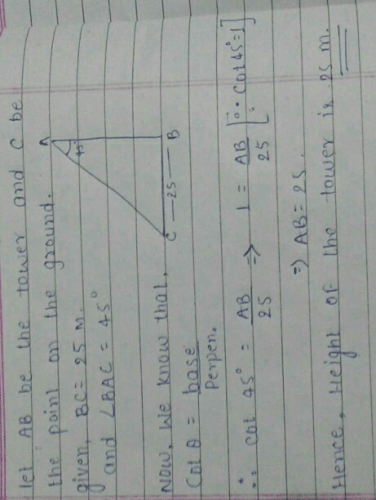A tower stands vertically on the ground. From...

### Related TestA tower stands vertically on the ground. From a point on the ground which is 25 m away from the foot of the tower, the angle of elevation of the top of the tower is found to be 45o. Then the height (in meters) of the tower is​
• a)
25
• b)
25√3
• c)
12.5
• d)
25√2Dronacharya InstituteA point on the ground which is 25 m away from the foot of the tower i. BC= 25 m
Let the height of the tower be x
The angle of elevation of the tower is found to be 45 degree.i.e.∠ACB=45°
In ΔABC
Using trigonometric ratiosHence the height of the tower is 25 m.

 1 Crore+ students have signed up on EduRev. Have you?Entangled Mystery.
A tower stands vertically on the ground. From a point on the ground which is 25 m away from the foot of the tower, the angle of elevation of the top of the tower is found to be 45o. Then the height (in meters) of the tower is​a)25b)25√3c)12.5d)25√2Correct answer is option 'A'. Can you explain this answer?View courses related to this question Explore Class 10 courses
 Explore Class 10 coursesView courses related to this questionAisha Negi
•••hey look , we know the distance from the foot of the tower is 25 m which means , B= 25m and the the angle of elevation is 45 degree •••

we need to find the height of the tower means P

then we know that tan theta = P/B

and tan 45 = P/ 25. ...
then 1= P/25 ...( becoz the value of tan 45 degree is 1)

P = 25 m ans••

•• that's it•••Afrin Shah
A tower stands vertically on the ground. From a point on the ground which is 25 m away from the foot of the tower, the angle of elevation of the top of the tower is found to be 45o. Then the height (in meters) of the tower is​a)25b)25√3c)12.5d)25√2Correct answer is option 'A'. Can you explain this answer?
Let us consider the h of the tower to be AB and the point on the ground is C which is 25 m away from the foot of the tower

tan 45 degree=1
AB/BC=1
AB=BC
AB=25m

hence ,,
is ur ans

Question Description
A tower stands vertically on the ground. From a point on the ground which is 25 m away from the foot of the tower, the angle of elevation of the top of the tower is found to be 45o. Then the height (in meters) of the tower is​a)25b)25√3c)12.5d)25√2Correct answer is option 'A'. Can you explain this answer? for Class 10 2023 is part of Class 10 preparation. The Question and answers have been prepared according to the Class 10 exam syllabus. Information about A tower stands vertically on the ground. From a point on the ground which is 25 m away from the foot of the tower, the angle of elevation of the top of the tower is found to be 45o. Then the height (in meters) of the tower is​a)25b)25√3c)12.5d)25√2Correct answer is option 'A'. Can you explain this answer? covers all topics & solutions for Class 10 2023 Exam. Find important definitions, questions, meanings, examples, exercises and tests below for A tower stands vertically on the ground. From a point on the ground which is 25 m away from the foot of the tower, the angle of elevation of the top of the tower is found to be 45o. Then the height (in meters) of the tower is​a)25b)25√3c)12.5d)25√2Correct answer is option 'A'. Can you explain this answer?.
Solutions for A tower stands vertically on the ground. From a point on the ground which is 25 m away from the foot of the tower, the angle of elevation of the top of the tower is found to be 45o. Then the height (in meters) of the tower is​a)25b)25√3c)12.5d)25√2Correct answer is option 'A'. Can you explain this answer? in English & in Hindi are available as part of our courses for Class 10. Download more important topics, notes, lectures and mock test series for Class 10 Exam by signing up for free.
Here you can find the meaning of A tower stands vertically on the ground. From a point on the ground which is 25 m away from the foot of the tower, the angle of elevation of the top of the tower is found to be 45o. Then the height (in meters) of the tower is​a)25b)25√3c)12.5d)25√2Correct answer is option 'A'. Can you explain this answer? defined & explained in the simplest way possible. Besides giving the explanation of A tower stands vertically on the ground. From a point on the ground which is 25 m away from the foot of the tower, the angle of elevation of the top of the tower is found to be 45o. Then the height (in meters) of the tower is​a)25b)25√3c)12.5d)25√2Correct answer is option 'A'. Can you explain this answer?, a detailed solution for A tower stands vertically on the ground. From a point on the ground which is 25 m away from the foot of the tower, the angle of elevation of the top of the tower is found to be 45o. Then the height (in meters) of the tower is​a)25b)25√3c)12.5d)25√2Correct answer is option 'A'. Can you explain this answer? has been provided alongside types of A tower stands vertically on the ground. From a point on the ground which is 25 m away from the foot of the tower, the angle of elevation of the top of the tower is found to be 45o. Then the height (in meters) of the tower is​a)25b)25√3c)12.5d)25√2Correct answer is option 'A'. Can you explain this answer? theory, EduRev gives you an ample number of questions to practice A tower stands vertically on the ground. From a point on the ground which is 25 m away from the foot of the tower, the angle of elevation of the top of the tower is found to be 45o. Then the height (in meters) of the tower is​a)25b)25√3c)12.5d)25√2Correct answer is option 'A'. Can you explain this answer? tests, examples and also practice Class 10 tests.

• ### A TV tower stands vertically on a bank of canal . from a point on the other... more(Scan QR code)